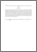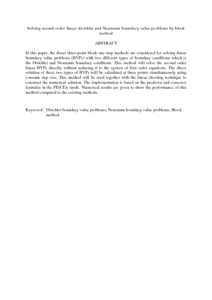# Solving second order linear dirichlet and Neumann boundary value problems by block method

## Citation

Abdul Majid, Zanariah and Hasni, Mohd Mughti and Senu, Norazak (2013) Solving second order linear dirichlet and Neumann boundary value problems by block method. IAENG International Journal of Applied Mathematics, 43 (2). pp. 71-76. ISSN 1992-9978; ESSN:1992-9986

## Abstract

In this paper, the direct three-point block one-step methods are considered for solving linear boundary value problems (BVPs) with two different types of boundary conditions which is the Dirichlet and Neumann boundary conditions. This method will solve the second order linear BVPs directly without reducing it to the system of first order equations. The direct solution of these two types of BVPs will be calculated at three points simultaneously using constant step size. This method will be used together with the linear shooting technique to construct the numerical solution. The implementation is based on the predictor and corrector formulas in the PE(CE)r mode. Numerical results are given to show the performance of this method compared to the existing methods.Preview
PDF (Abstract)
Solving second order linear dirichlet and Neumann boundary value problems by block method.pdfView Item